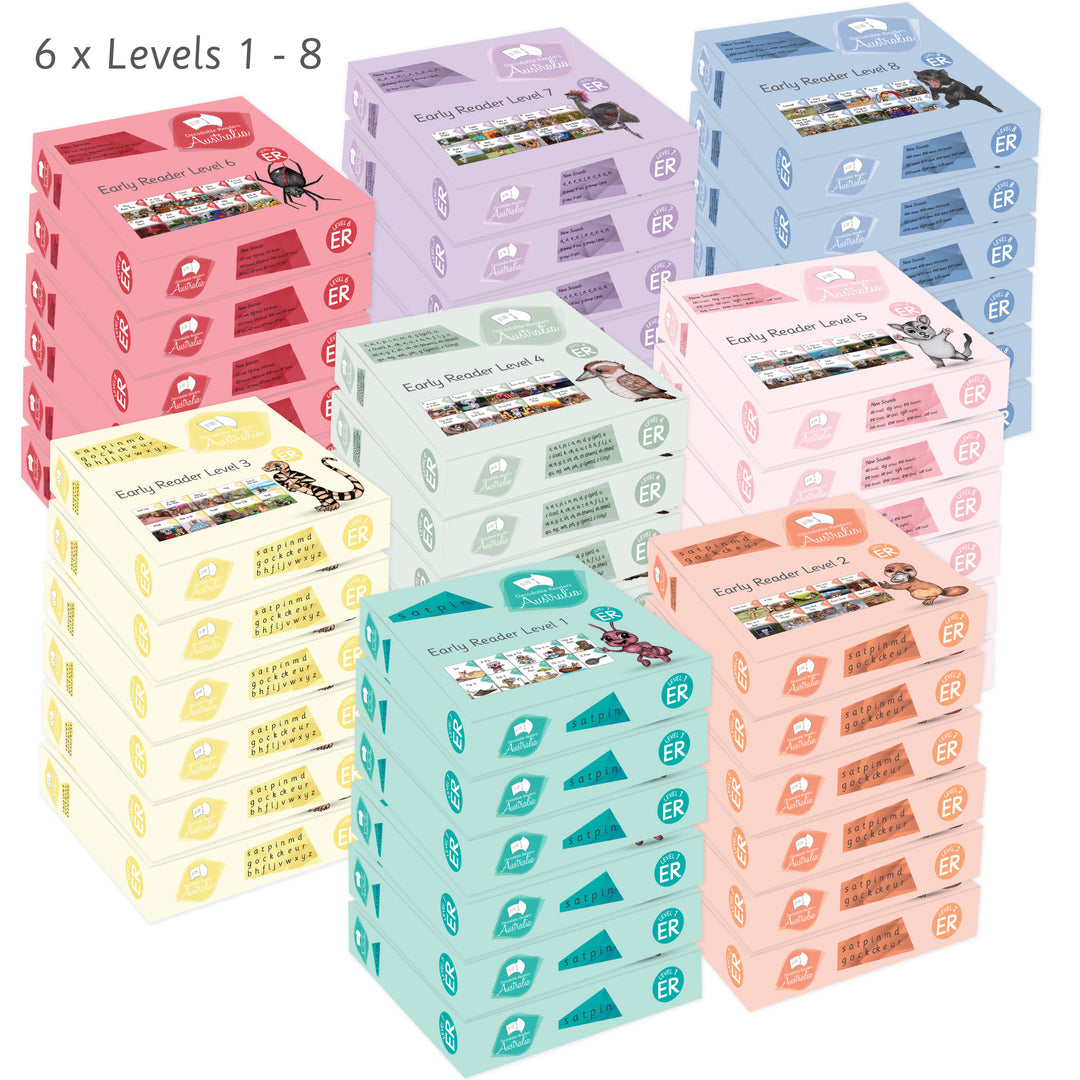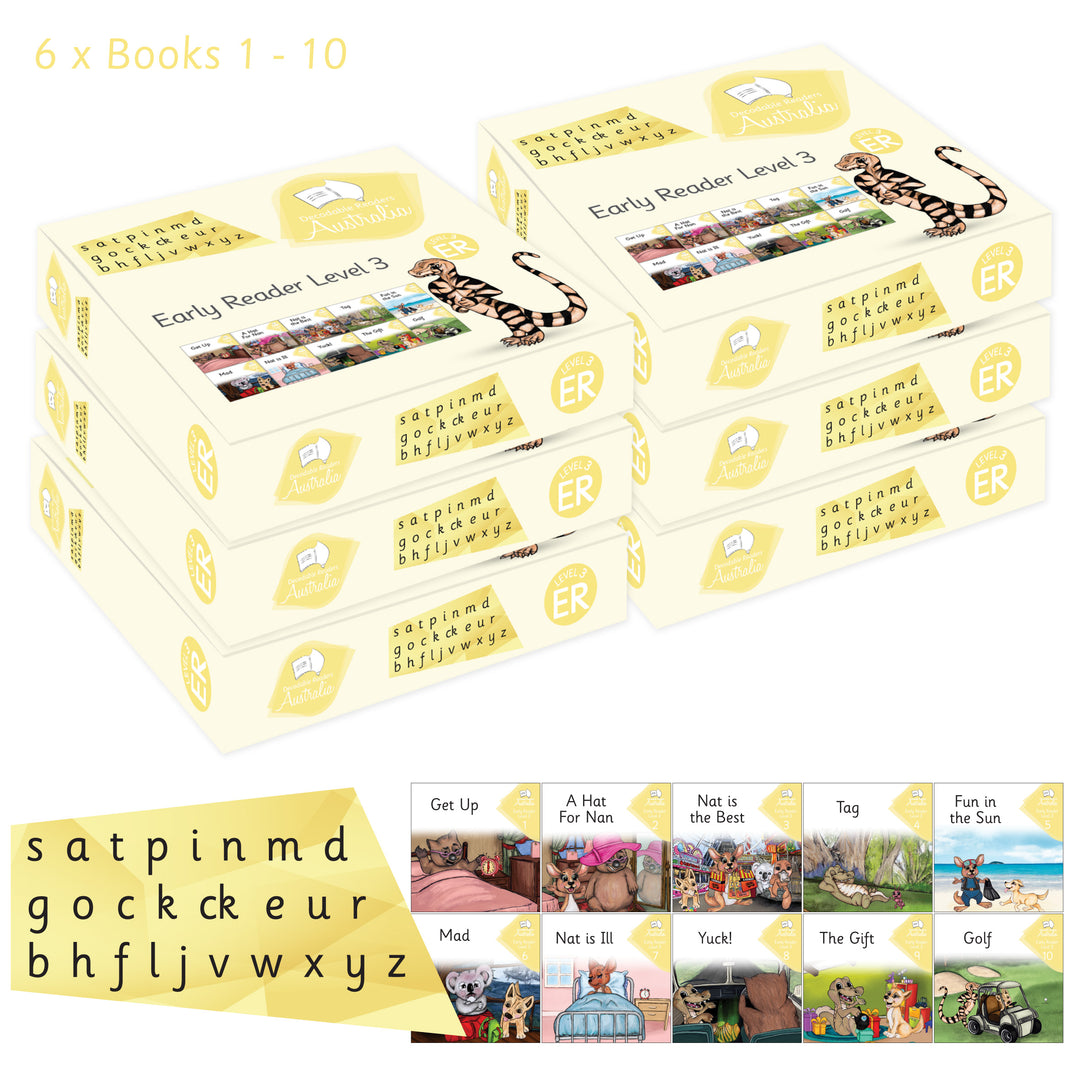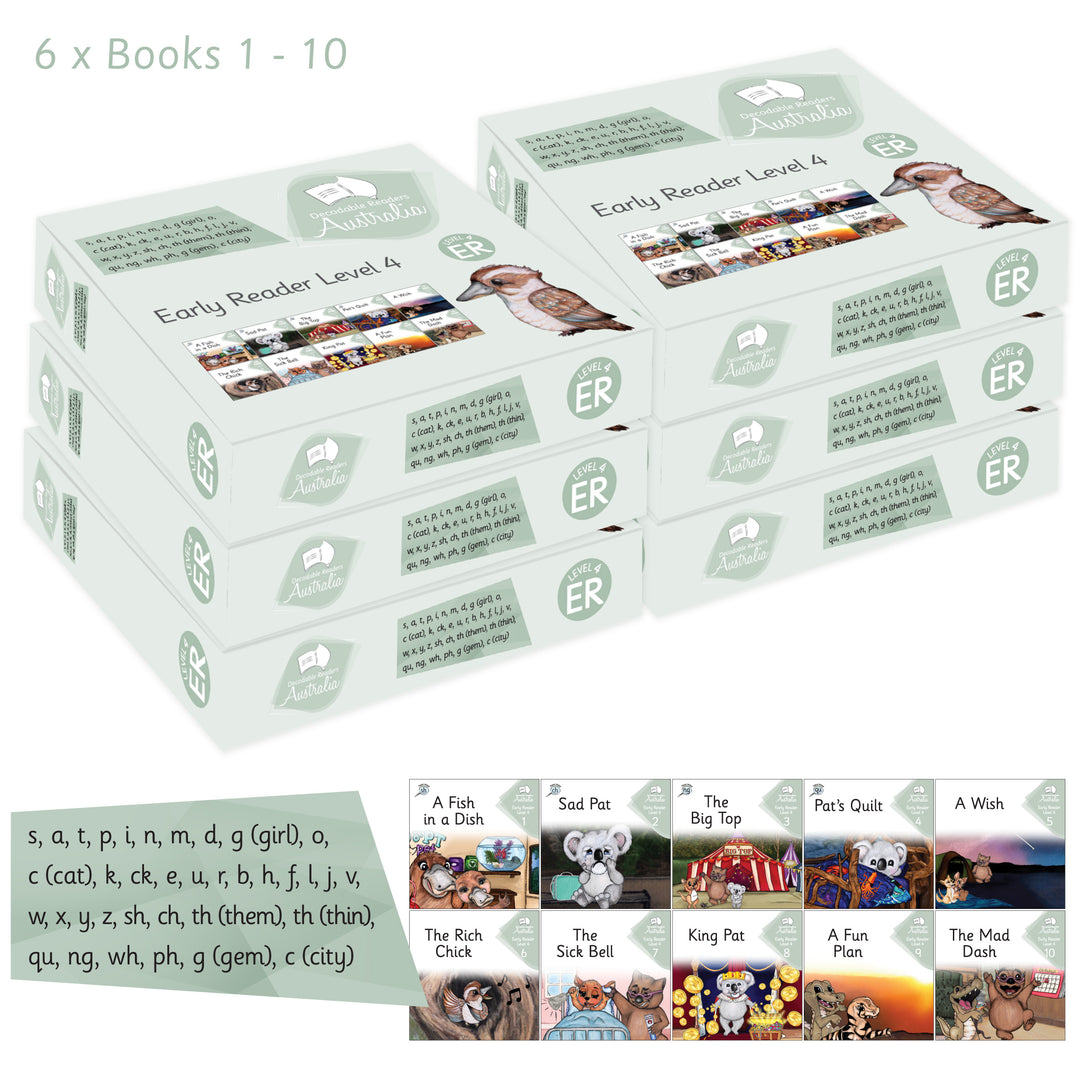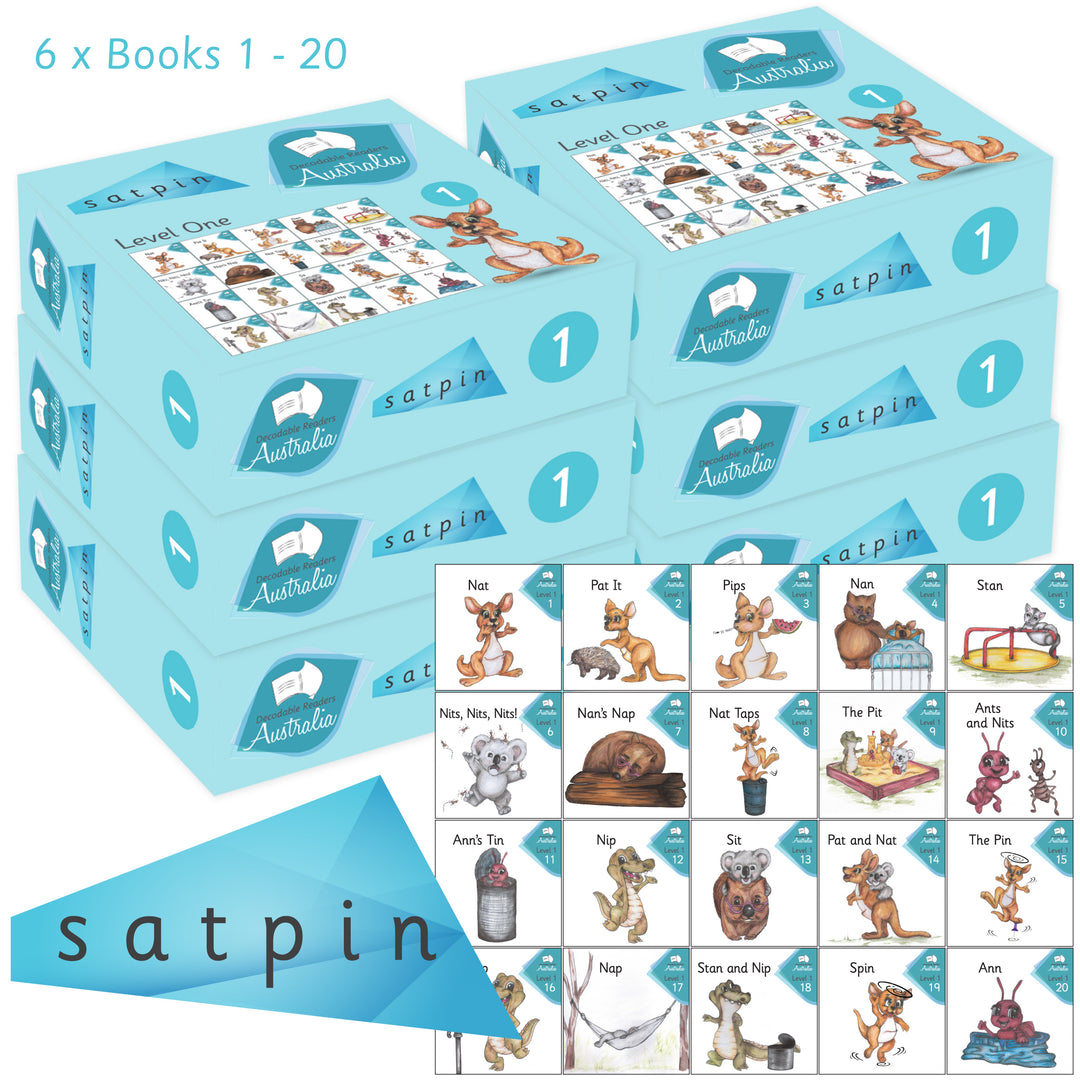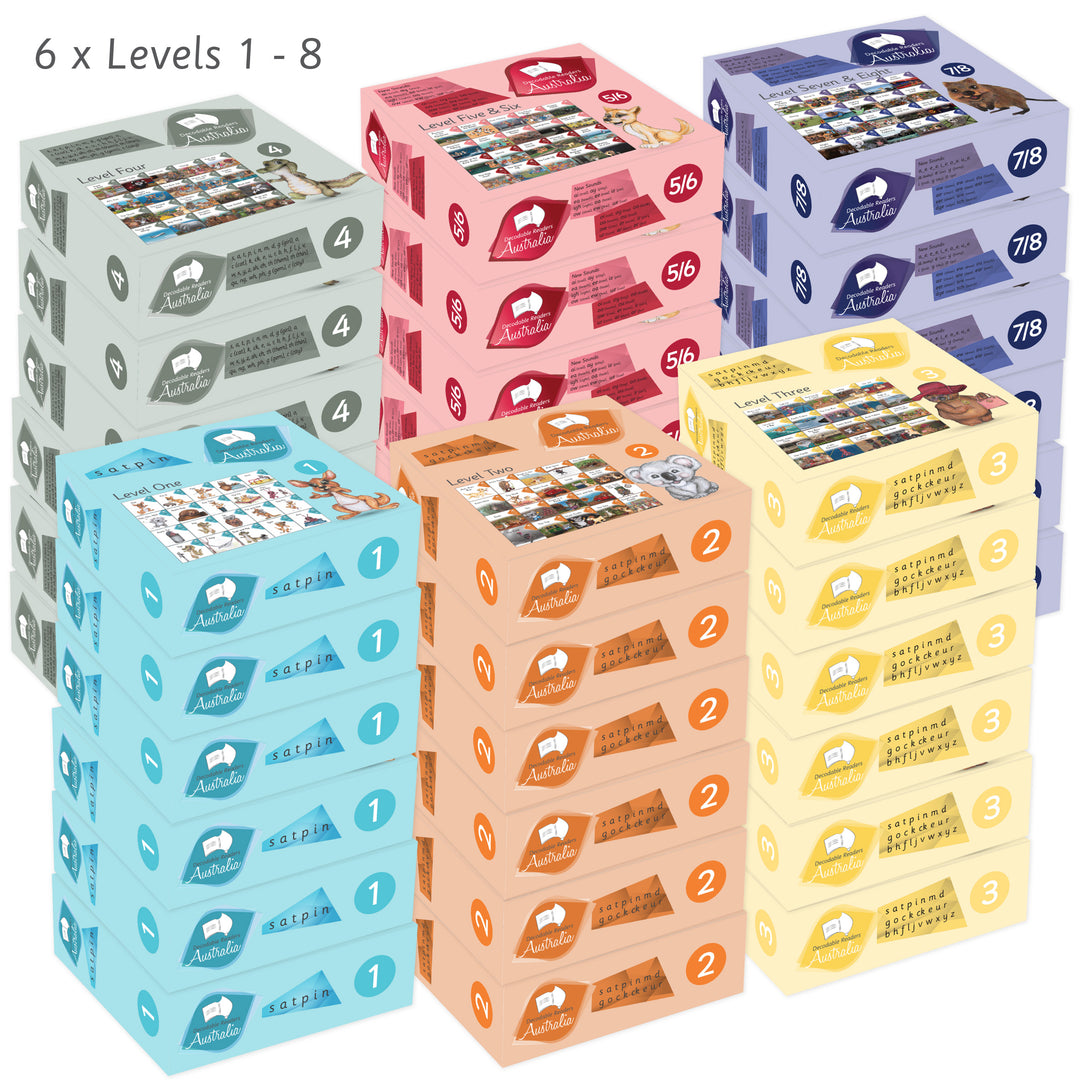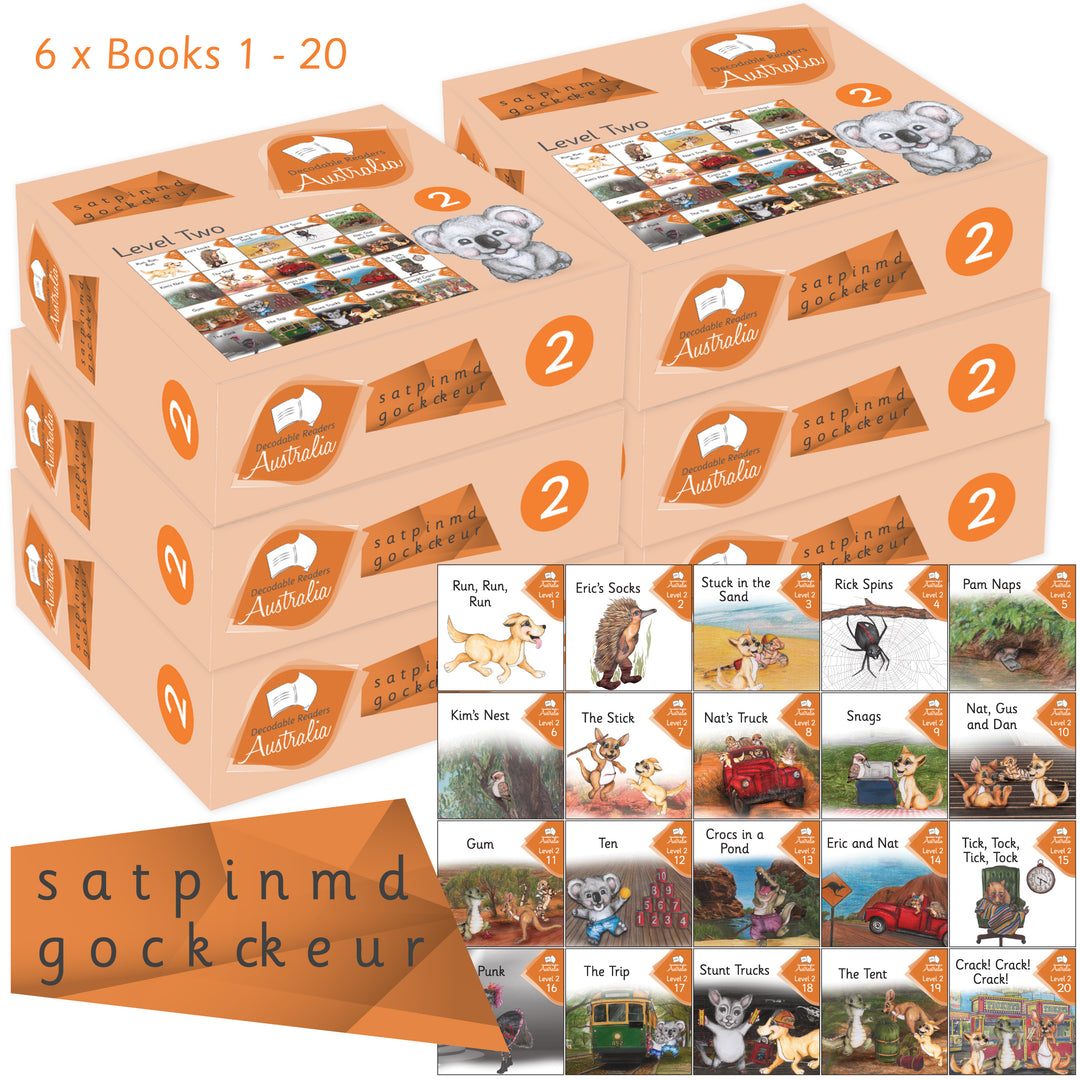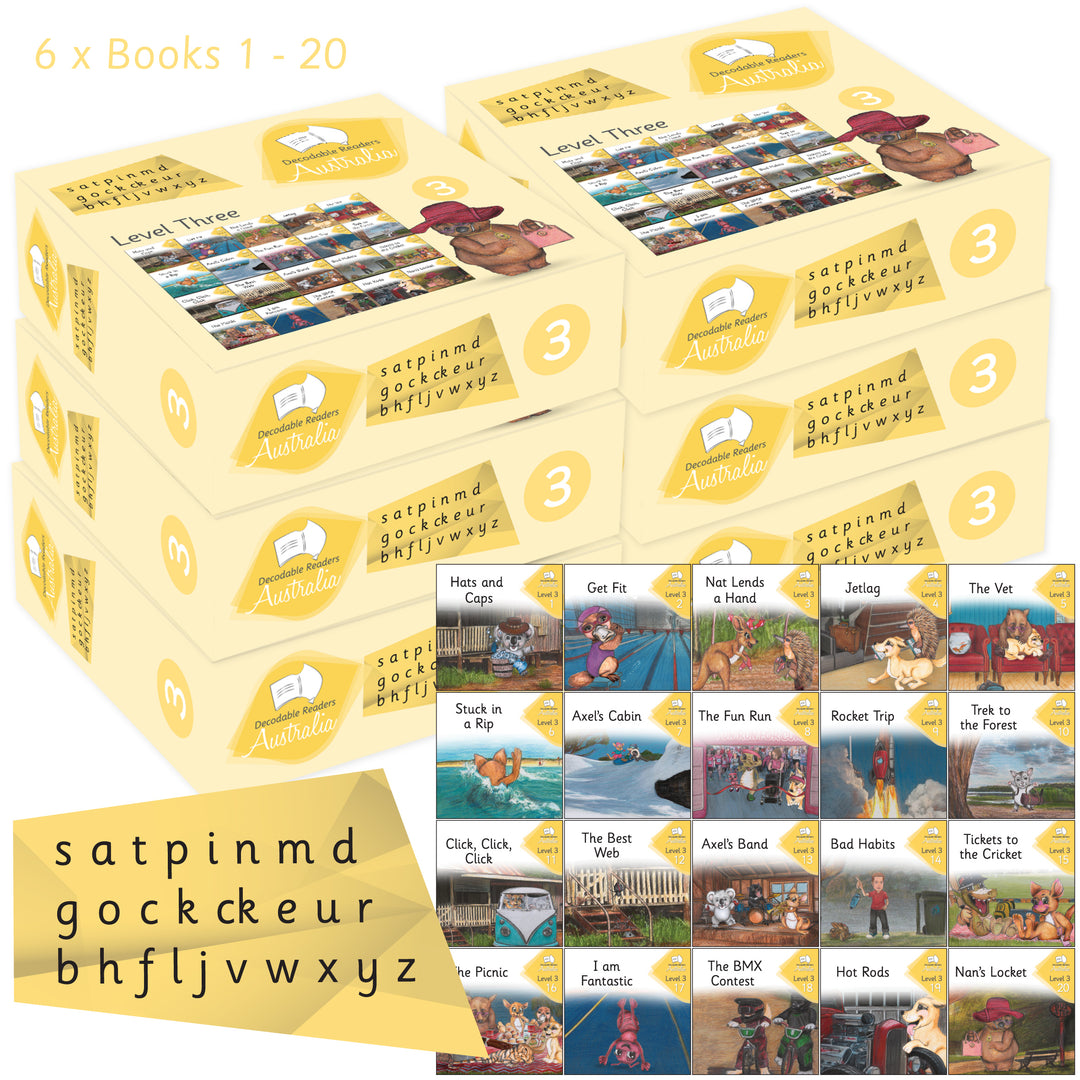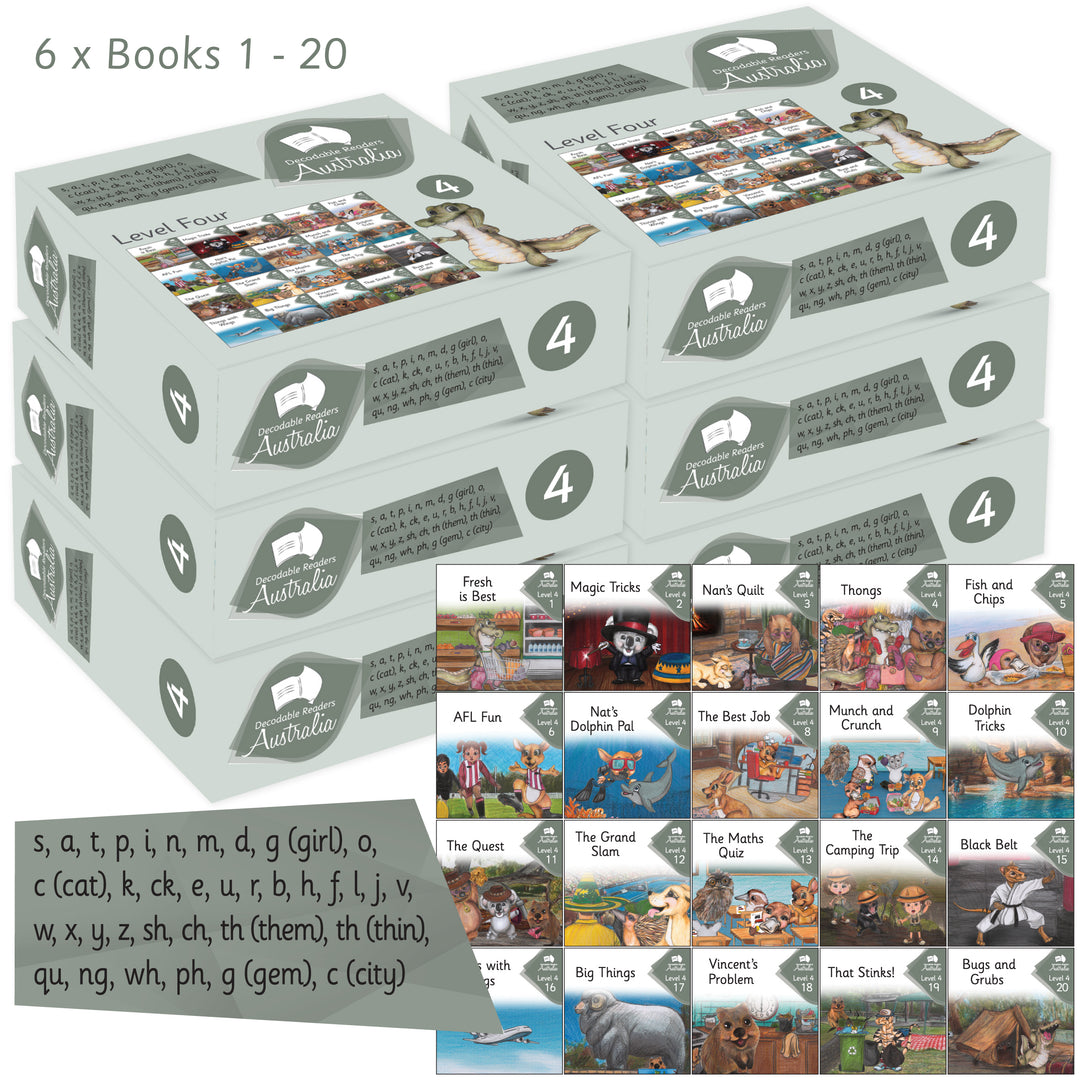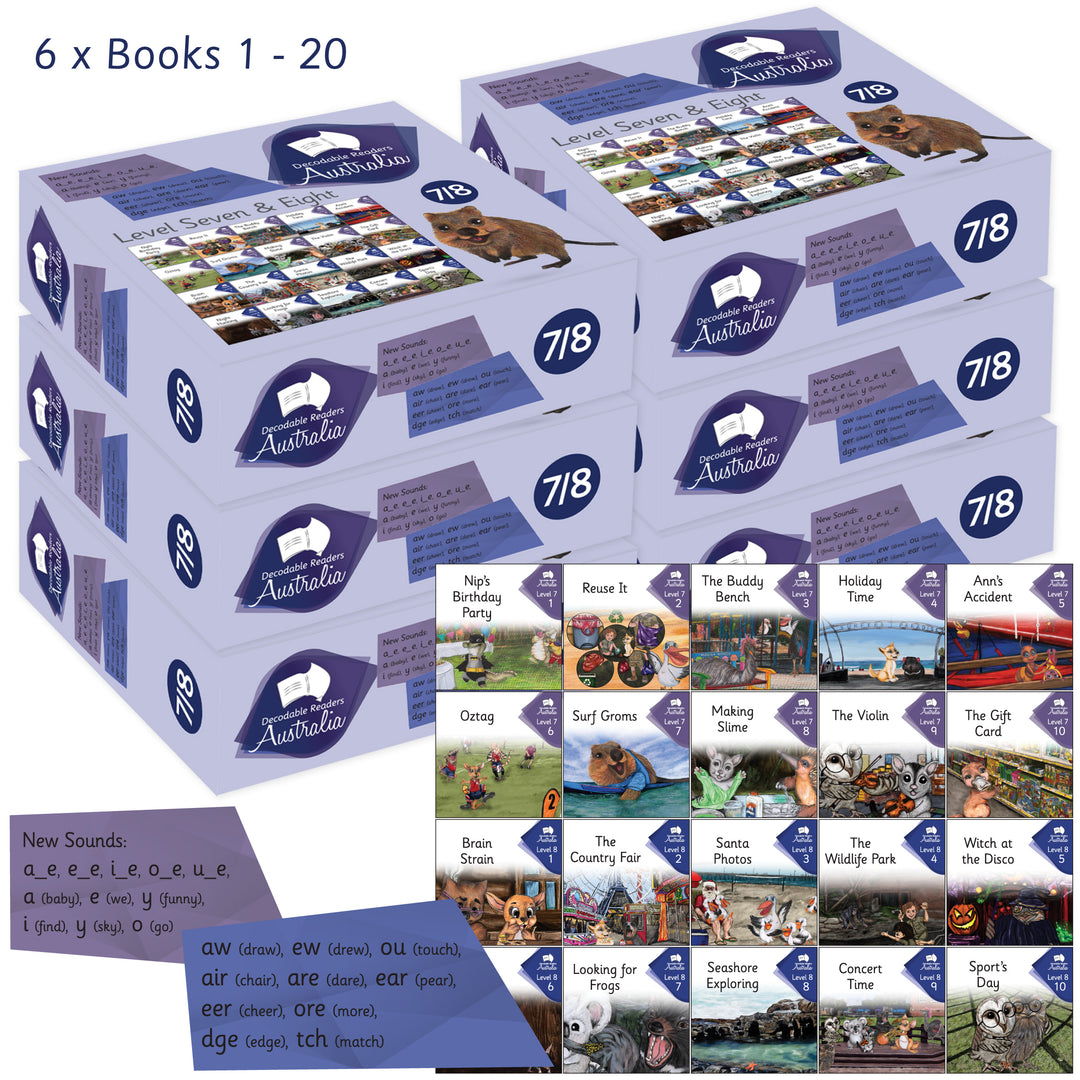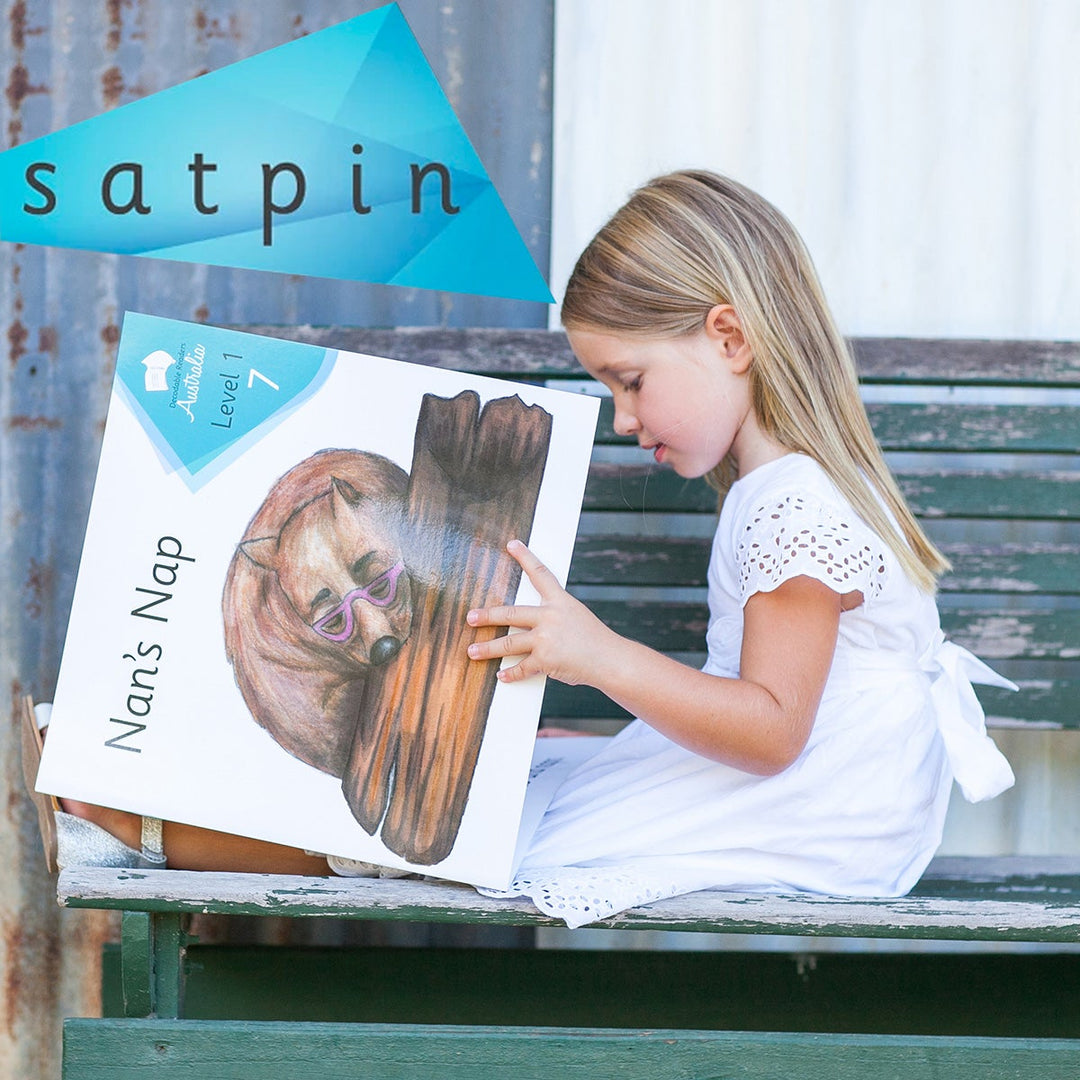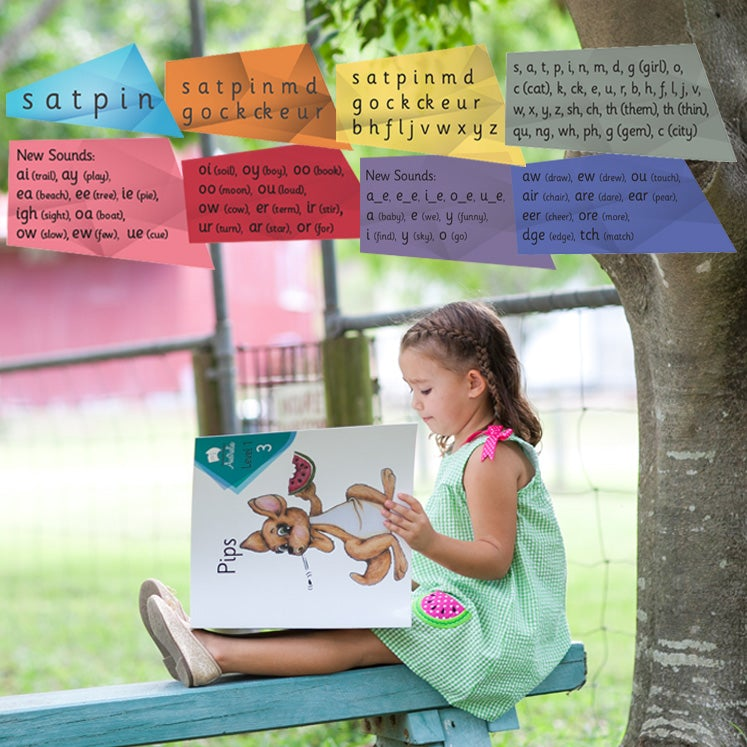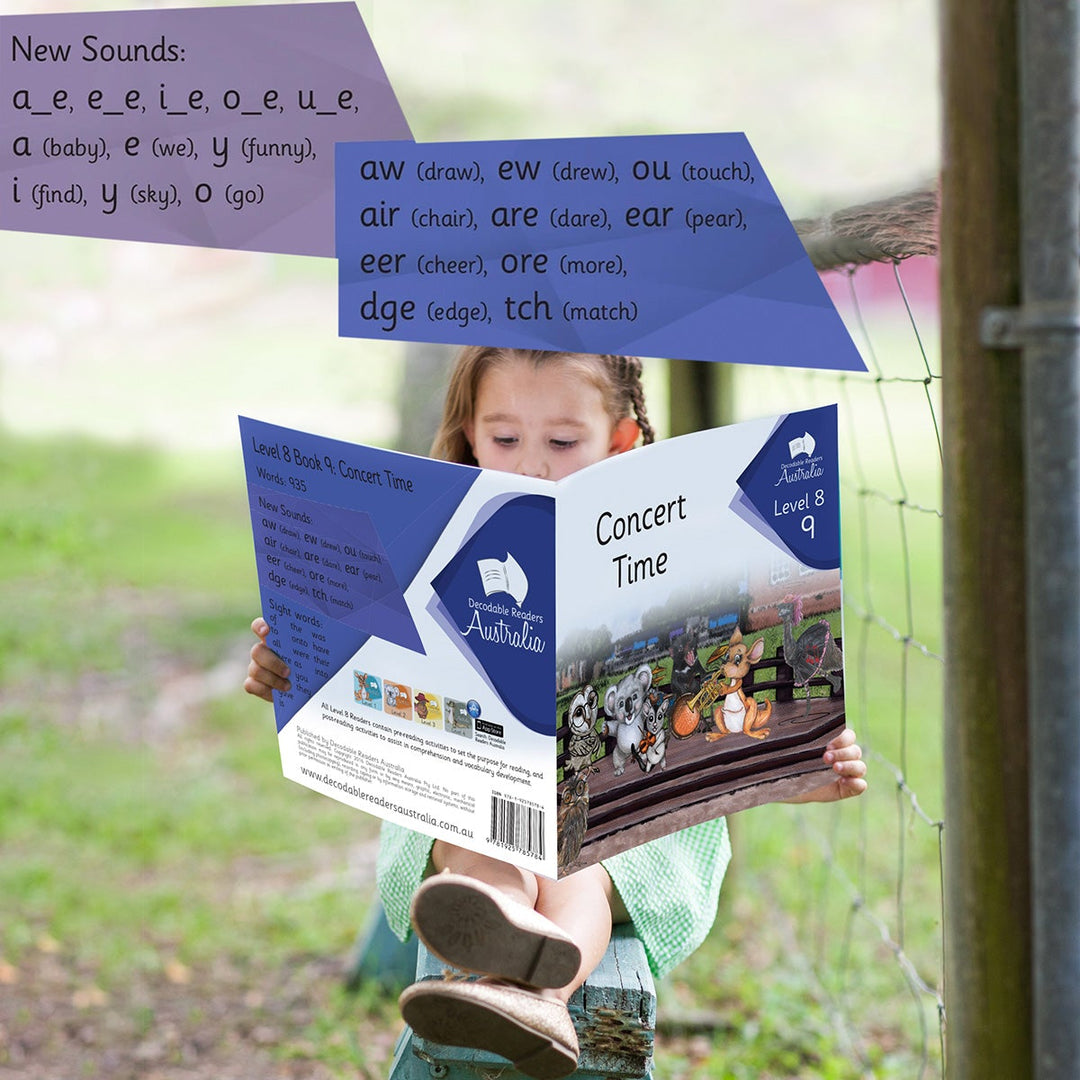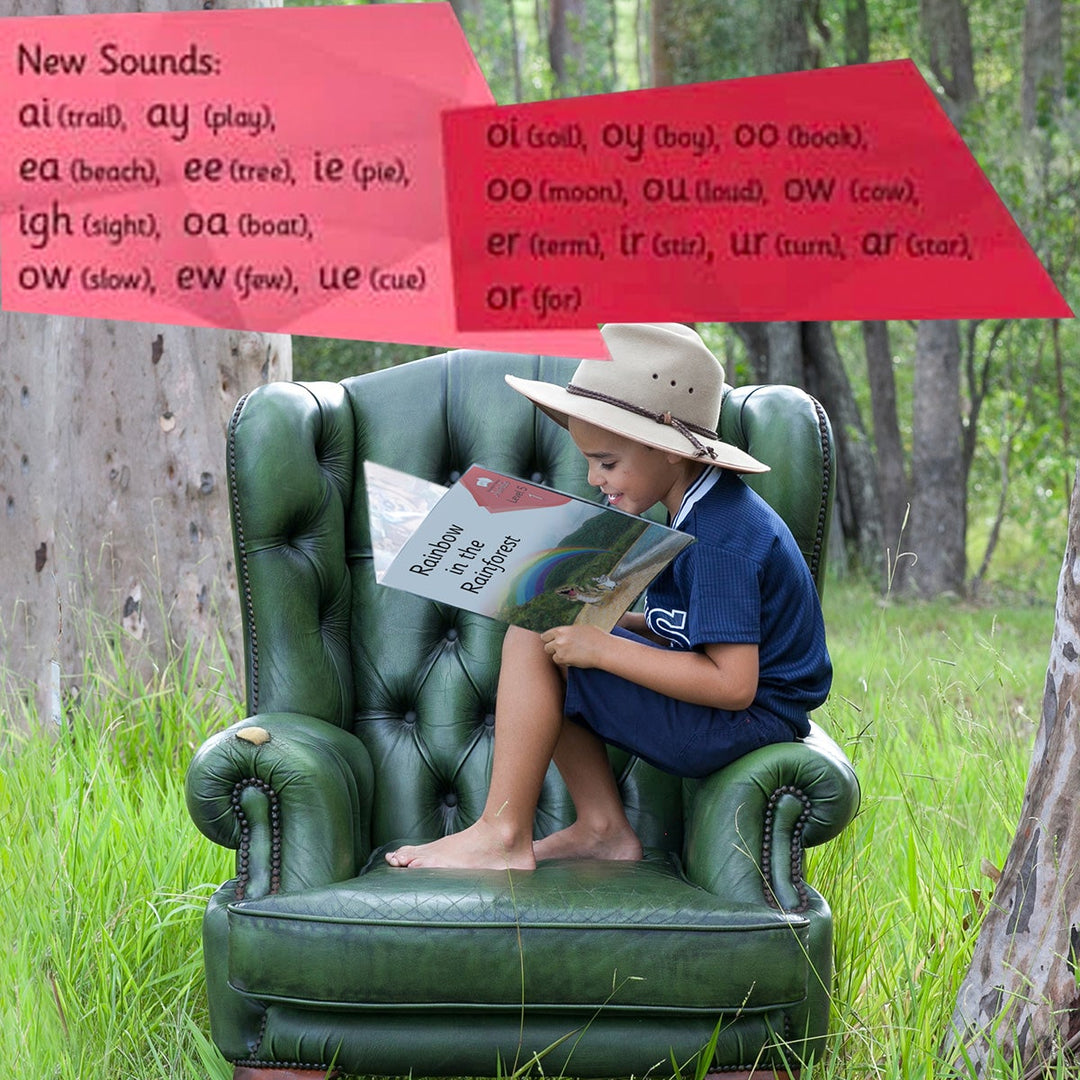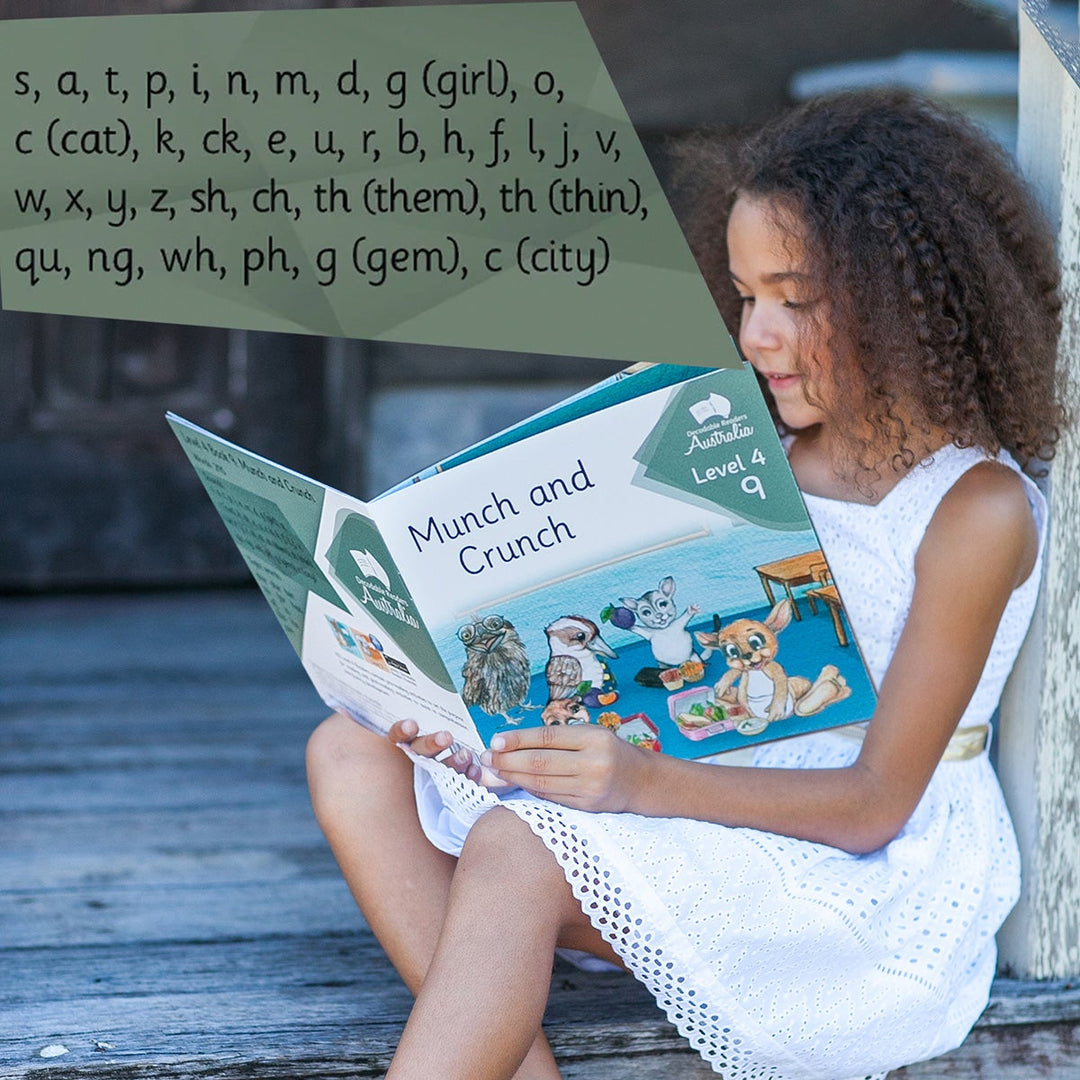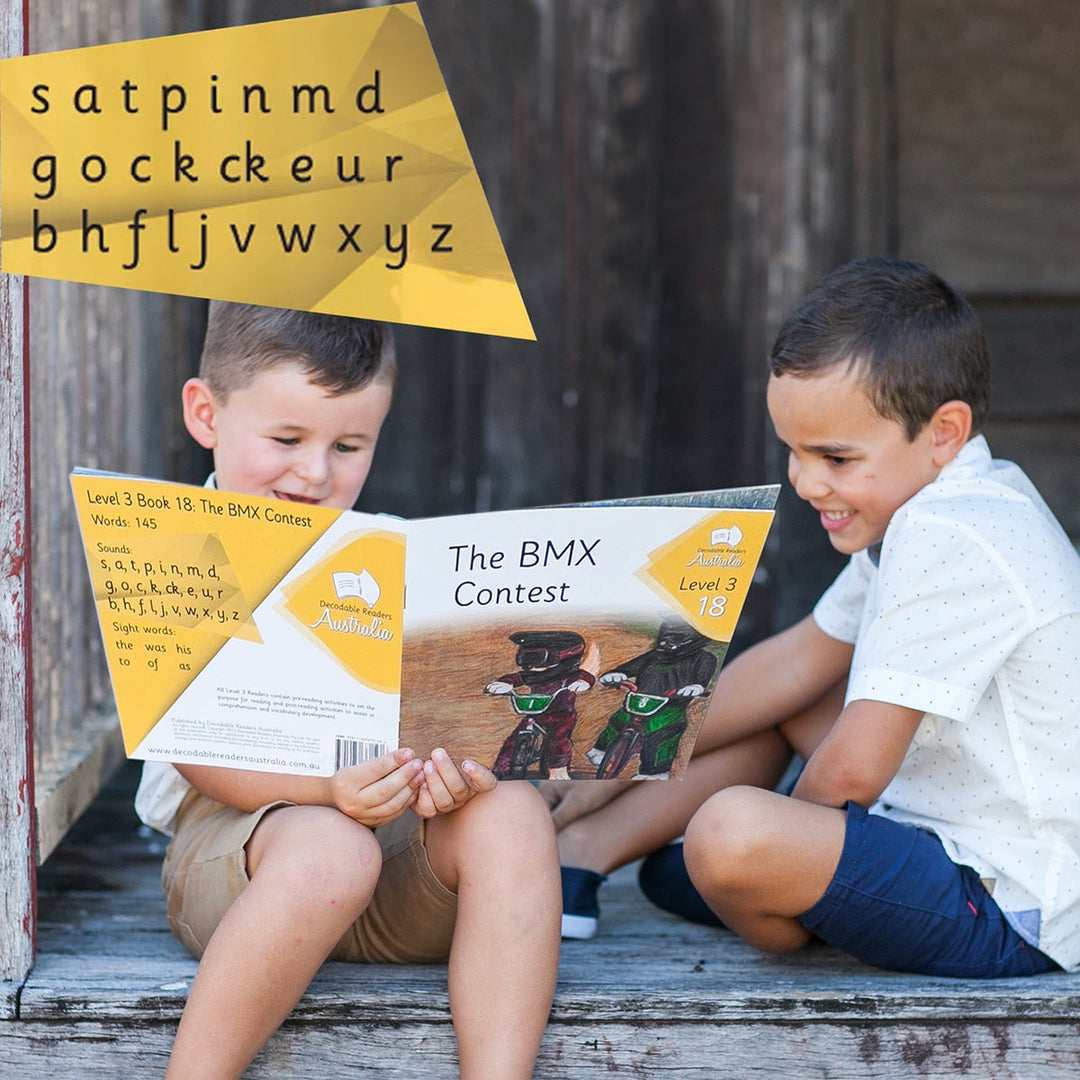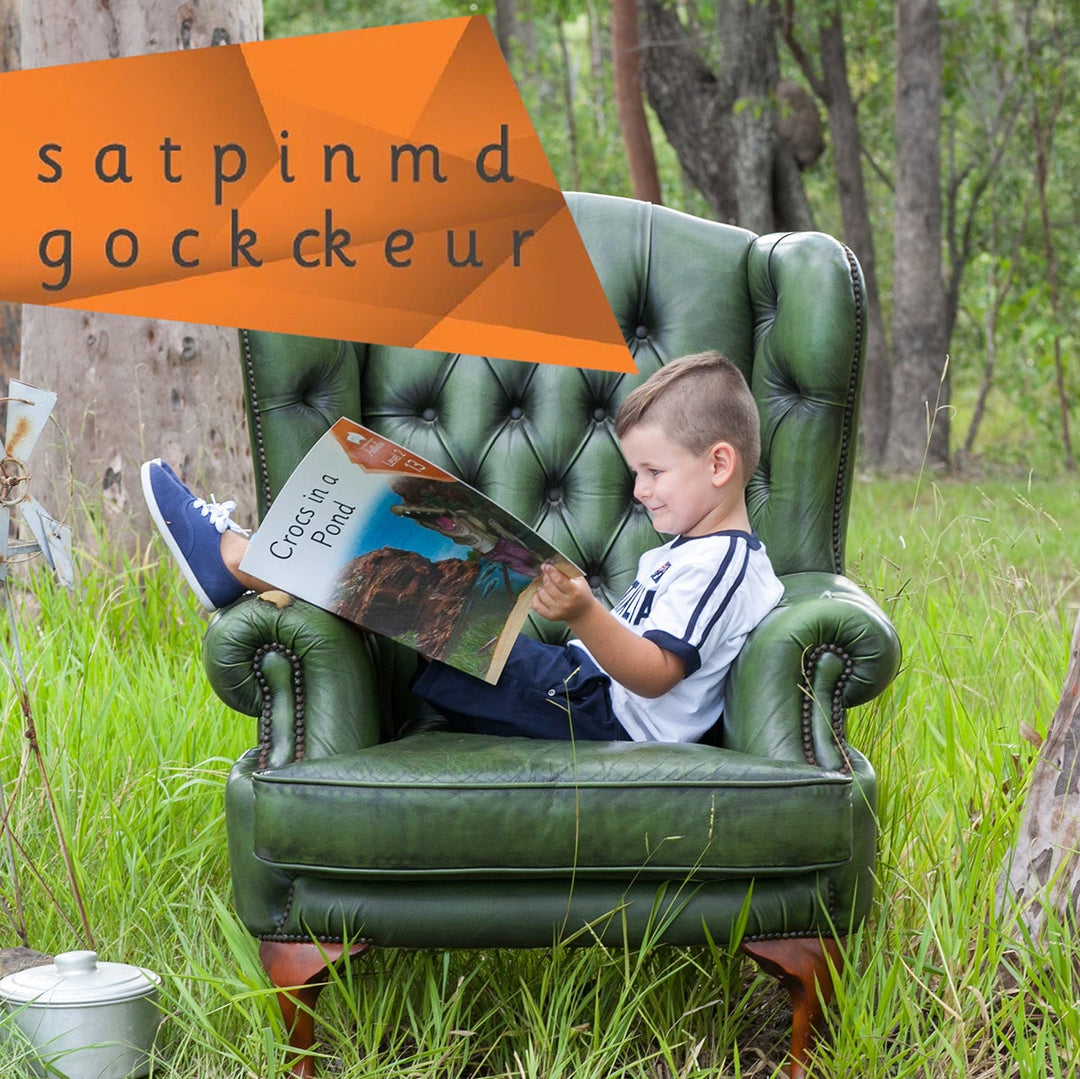# Bulk Resource Pack 2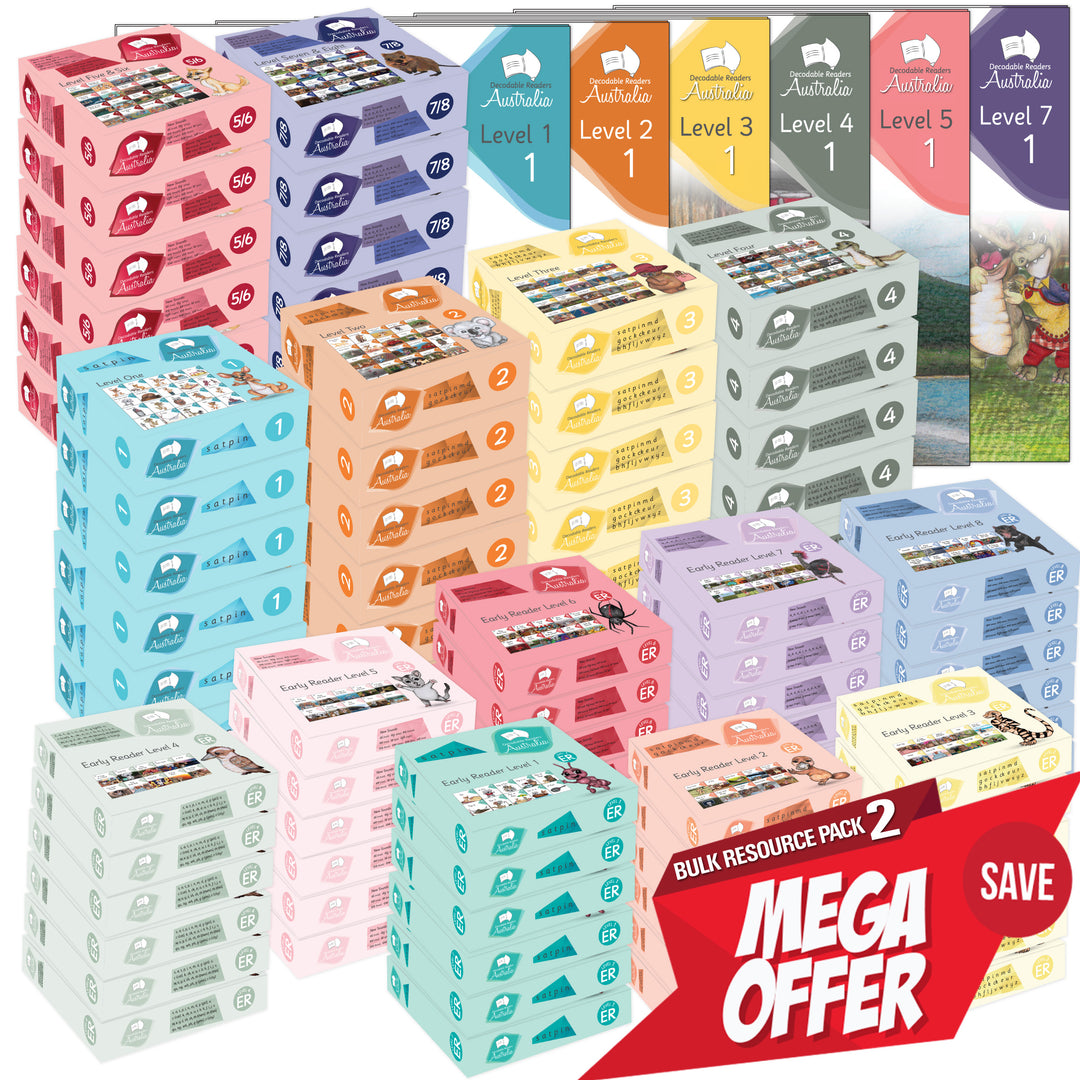\$7,995.00 AUD

\$9,276.00 AUD

Bulk Resource Pack 2
Levels 1 to 8

Resource your Early Years Classrooms, Learning Support and Library!

6 x Complete individual Early Readers Sets =
6x Level 1 = 60 Books
6x Level 2 = 60 Books
6x Level 3 = 60 Books
6x Level 4 = 60 Books
6x Level 5 = 60 Books
6x Level 6 = 60 Books
6x Level 7 = 60 Books
6x Level 8 = 60 Books

= 480 Books

6 x Complete individual Set =
6x Level 1 = 120 Books
6x Level 2 = 120 Books
6x Level 3 = 120 Books
6x Level 4 = 120 Books
6x Level 5/6 = 120 Books
6x Level 7/8 = 120 Books
= 720 Books

+ Complete Big Books Set =
1x Level 1 = 20 Big Books
1x Level 2 = 20 Big Books
1x Level 3 = 20 Big Books
1x Level 4 = 20 Big Books
1x Level 5/6 = 20 Big Books
1x Level 7/8 = 20 Big Books
= 120 Big Books